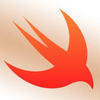# Recap: Function 3/3

func temperatureInFahrenheit(temperature: Double) -> Double {

```return(temperature * 9) / 5 + 32
```

}

temperatureInFahrenheit(temperature: 24.0)

let temperatureInFahrenheit(temperature: Double) = fahrenheitTemp

//call the function and pass in a value of 24.0 degrees. Assign the result of the function to a

constant named fahrenheitTemp.

//Why does this code not take? I feel its the simplest thing but I just can't get it.

functions.swift
```func temperatureInFahrenheit(temperature: Double) -> Double {

return(temperature * 9) / 5 + 32

}

temperatureInFahrenheit(temperature: 24.0)

let farenheitTemp = temperatureInFahrenheit(temperature: <#T##Double#>)
```Not sure why you are including the last part of your code

```func temperatureInFahrenheit(temperature: Double) -> Double {

return temperature * 9 / 5 + 32

}

let fahrenheitTemp = temperatureInFahrenheit(temperature: 24.0)
```Hey Jeff,

I followed the video and wrote my code a different way and was still able to pass. Can you help answer the following question?

func temperatureInFahrenheit (temperature: Double) -> Double { let fahrenheit = temperature * 9 / 5 + 32 return (fahrenheit) } let fahrenheitTemp = temperatureInFahrenheit (temperature: 24.0)

After looking at your code, I noticed I created an extra constant "fahrenheit", if I did that does that mean the last statement is passing in the value of constant "fahrenheit" into "fahrenheitTemp" where yours is just passing in the result of the calculation into "fahrenheitTemp"? Thanks!Yes you are correct you basically created a new variable which is ok but not needed

See some Apple documentation

Here they created the variable greeting

```func greet(person: String) -> String {
let greeting = "Hello, " + person + "!"
return greeting
}
```

but it is really not needed because you can just combine and use return

```func greetAgain(person: String) -> String {
return "Hello again, " + person + "!"
}
```

Let me know if that makes sense